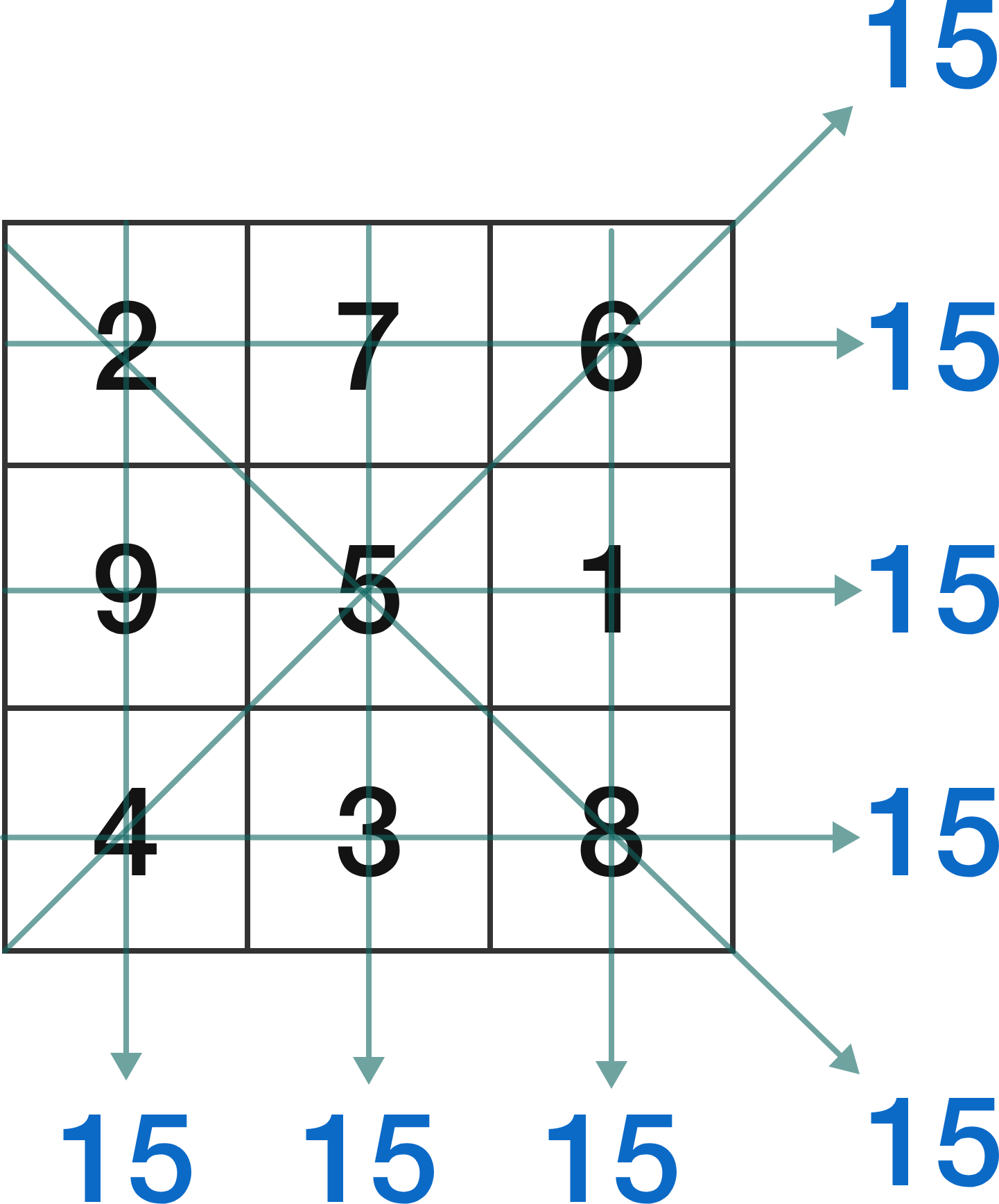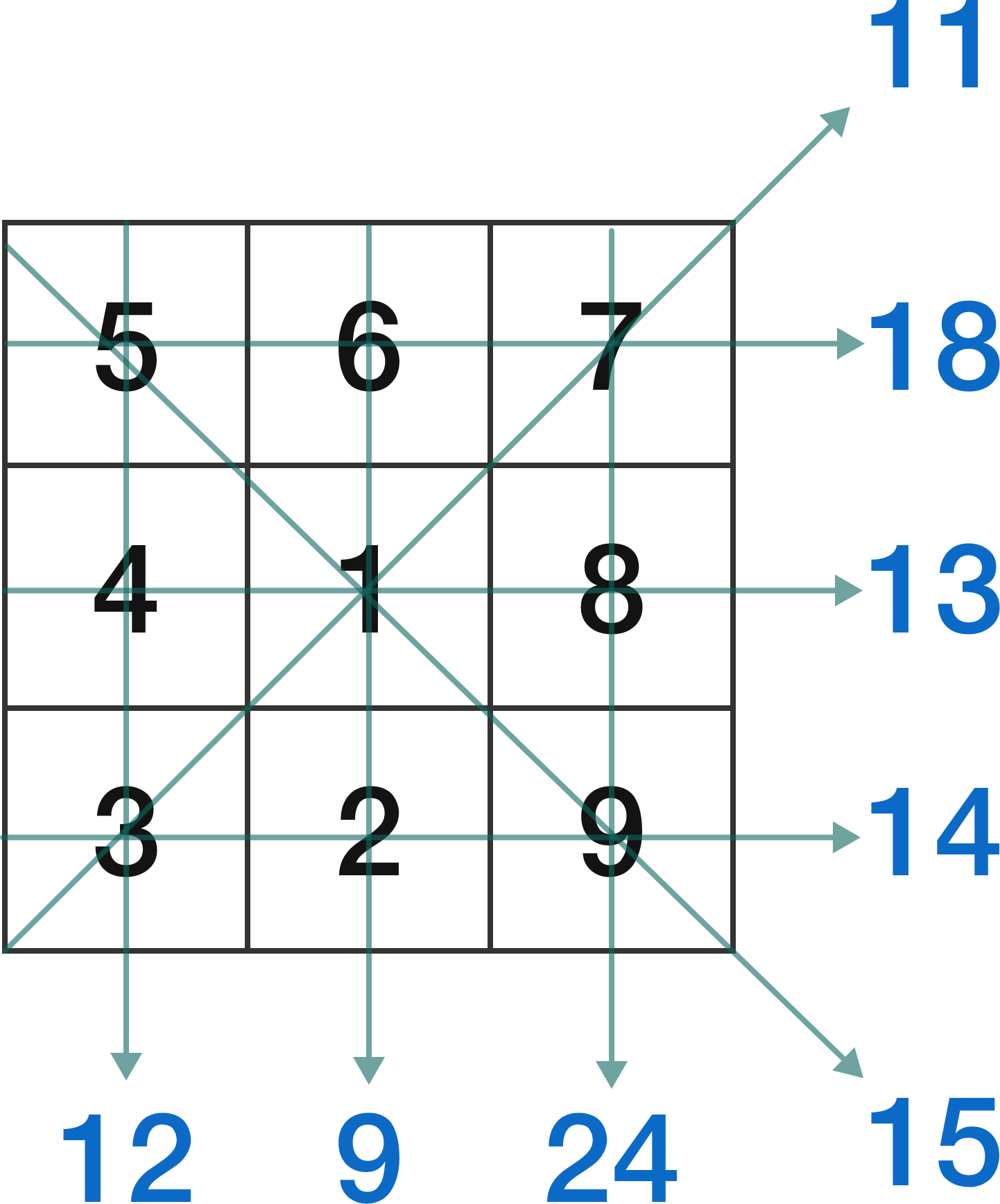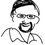# ===Open Problem #2=== (solved!)

A magic square contains positive consecutive integers starting from 1 such that the rows, columns, and diagonals all add to the same value.A heterosquare contains positive consecutive integers starting from 1 such that the rows, columns, and diagonals all add to different values.If the sums resulting from a heterosquare form a consecutive sequence, the heterosquare is also called an antimagic square. (In the example above, if the sums of 9 and 24 were 16 and 17 instead, it would be a antimagic square.)

Prove that there are no 3 by 3 antimagic squares.

Yes, we have a proof! Thanks to: Mike Harding, Steven Jim, Marcus Luebke, Stefan Van der Waal, and Steven Yuan. (I believe that's everyone who contributed - please chime in if I missed someone.)

Now, there still are quite possibly improvements to be made, so even if you haven't contributed yet, feel free to check the proof at the wiki to see if any of the steps can be condensed. It's particularly useful to do so in this case because there was already a brute-force computer proof of the above fact; therefore it would be nice to have the most elegant proof possible.

Fairly soon (starting in two weeks) I will put the text down in a LaTeX file, add an introduction and citations, and work on getting it published in an actual journal.

Open Problem #3 will start January 8th.Note by Jason Dyer
3 years, 3 months ago

This discussion board is a place to discuss our Daily Challenges and the math and science related to those challenges. Explanations are more than just a solution — they should explain the steps and thinking strategies that you used to obtain the solution. Comments should further the discussion of math and science.

When posting on Brilliant:

• Use the emojis to react to an explanation, whether you're congratulating a job well done , or just really confused .
• Ask specific questions about the challenge or the steps in somebody's explanation. Well-posed questions can add a lot to the discussion, but posting "I don't understand!" doesn't help anyone.
• Try to contribute something new to the discussion, whether it is an extension, generalization or other idea related to the challenge.
• Stay on topic — we're all here to learn more about math and science, not to hear about your favorite get-rich-quick scheme or current world events.

MarkdownAppears as
*italics* or _italics_ italics
**bold** or __bold__ bold
- bulleted- list
• bulleted
• list
1. numbered2. list
1. numbered
2. list
Note: you must add a full line of space before and after lists for them to show up correctly
paragraph 1paragraph 2

paragraph 1

paragraph 2

[example link](https://brilliant.org)example link
> This is a quote
This is a quote
    # I indented these lines
# 4 spaces, and now they show
# up as a code block.

print "hello world"
# I indented these lines
# 4 spaces, and now they show
# up as a code block.

print "hello world"
MathAppears as
Remember to wrap math in $$ ... $$ or $ ... $ to ensure proper formatting.
2 \times 3 $2 \times 3$
2^{34} $2^{34}$
a_{i-1} $a_{i-1}$
\frac{2}{3} $\frac{2}{3}$
\sqrt{2} $\sqrt{2}$
\sum_{i=1}^3 $\sum_{i=1}^3$
\sin \theta $\sin \theta$
\boxed{123} $\boxed{123}$

## Comments

Sort by:

Top Newest

The first part of the proof can be condensed.

We all know that $2(b+d+f+h)+3(a+c+g+i)+4e=8n+28$ or $a+c+g+i+2e=8n-62$. Also, the minimum and maximum values of $(c+g+e)+(a+i+e)$ are $2n+1$ and $2n+15$, respectively, which means $2n+1 \le 8n-62 \le 2n+15$ or $11 \le n \le 12$ (as $n$ must be an integer.

That's it.

- 3 years, 3 months ago

Log in to reply

×

Problem Loading...

Note Loading...

Set Loading...